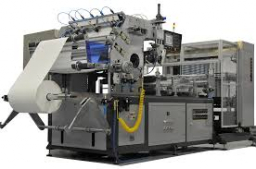# Calculate 38563

Six equally powerful machines produce 870 parts in 14 hours. Calculate in how many hours 870 parts will have four such machines.

t2 =  21 h

### Step-by-step explanation:Did you find an error or inaccuracy? Feel free to write us. Thank you!

Tips for related online calculators
Do you have a linear equation or system of equations and looking for its solution? Or do you have a quadratic equation?
Do you want to convert time units like minutes to seconds?